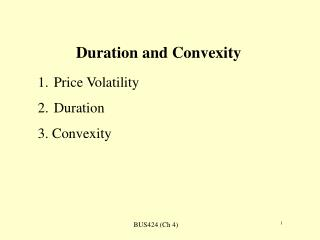DownloadDownload PresentationDuration and Convexity

# Duration and Convexity

Download Presentation## Duration and Convexity

- - - - - - - - - - - - - - - - - - - - - - - - - - - E N D - - - - - - - - - - - - - - - - - - - - - - - - - - -
##### Presentation Transcript

1. Duration and Convexity • Price Volatility • Duration • 3. Convexity

2. Price Volatility of Different Bonds Why do we have this chapter? -- how bond price reacts to changes in bond characteristics, including the following: -- Coupon rate: Inversely related to price volatility. -- Term to maturity: Positively related to price volatility. -- Yield to maturity: Inversely related to price volatility.

3. Measures of Price Volatility (1) • (A) Price value of a basis point • also known as dollar value of an 01. It is the change of bond price when required yield is changes by 1 bp. • Example: see page 62 • 5-year 9% coupon, YTM=9%, initial price=100, Price at 9.01% is _____; price value of a basis point = Abs(price change) =

4. Measures of Price Volatility (2) • (B) Yield value of a price change • change in the yield for a specified price change. • the smaller the number, the larger price volatility.

5. Example for Yield value of a price change • Maturity Ini yld Ini Prc Prc Chg Yld Chg • 5 years 100 9% 1 ? • 10 years 100 9% 1 ?

6. (c) Macaulay duration It can be shown that Where,

7. Modified Duration • Modified Duration=Macaulay duration/(1+y) Modified duration is a proxy for the percentage in price. It can be interpreted as the approximate percentage change in price for a 100-basis-point change in yield. Look at exhibit 4-12 on page 74: graphic interpretation of duration

8. Approximating the Dollar Price Change

9. Example • Calculate Macaulay duration and modified duration for a 5-year bond selling to yield 9% following the examples in the text. • What is the dollar change in bond price when interest rate increases by 1%?

10. Portfolio Duration • Find the weighted average duration of the bonds in the portfolios. • Contribution to portfolio duration: multiplying the weight of a bond in the portfolio by the duration of the bond. • See the example on pages 71-72.

11. Approximating a Bond’s Duration • Page 84

12. Convexity • Duration is only for small changes in yield or price – assuming a linear relation between yield change and price change • Convexity is for the nonlinear term • See the graph on page 75

13. Measuring Convexity • Taylor Series where

14. Example • Coupon rate: 6% • Term: 5 years • Initial yield: 9% • Price: 100 • (page 76)

15. Percentage Change in price due to Convexity Example: see page 76

16. Implications High convexity, high price, low yield. Convexity is valuable. Convexity is more valuable when market volatility is high. Look at Exhibit 4-12 on page 80.

17. Approximate Convexity Measure • Page 84

18. Percentage Price Change using Duration and Convexity Measure Example see page 78 - 79.

19. Yield risk for nonparallel changes in interest rates • 1). Yield Curve Reshaping Duration • Page 86: a) SEDUR; b) LEDUR • Formula for both are identical to 4.23 on page 84. • 2) Key rate duration (page 86) • Assume in every yield, we have a different duration. Then we have a duration vector.

20. Key rate durations • page 87 • Rate duration: the sensitivity of the change in value to a particular change in yield • Key rate durations (duration vectors) are measured using: 3m, 1 year, 2-year, 3-year, 5-year, 7-year, 10-year, 15-year, 20-year, 25-year and 30-year bonds